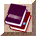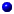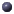##struct edv

Early drop variables, maintained by REDint count
of packets since last dropint count_bytes
of bytes since last dropedv()int old
0 when average queue first exceeds threshdouble v_a
v_prob = v_a * v_ave + v_bTracedDouble v_ave
average queue sizedouble v_bdouble v_c
used for "gentle" modedouble v_d
used for "gentle" modedouble v_prob
probTracedDouble v_prob1
probdouble v_slope
used in computing average queue size

## Documentation

Early drop variables, maintained by REDTracedDouble v_ave
average queue sizeTracedDouble v_prob1
prob. of packet drop before "count".double v_slope
used in computing average queue sizedouble v_prob
prob. of packet dropdouble v_a
v_prob = v_a * v_ave + v_bdouble v_bdouble v_c
used for "gentle" modedouble v_d
used for "gentle" modeint count
of packets since last dropint count_bytes
of bytes since last dropint old
0 when average queue first exceeds threshedv()